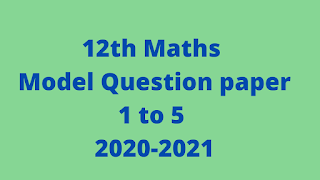#12th Maths Model Question paper

12th Maths Model Question paper based on reduced syllabus 2020-2021. 12th Standard Mathematics Reduced Syllabus Model Question Paper 2020-2021. Students can use this question papers. 12th maths model question paper based on public question pattern. Max Marks 90 time 3 hr. 10 marks for assessment and attendance record works. Class 12 Mathematics Model Question Paper. 12th maths,rbse 12th maths model paper 2021,12th maths model question paper 2021,12th maths reduced model question paper 2021,12th maths government model question paper 2021,cbse sample paper 2021 class 12 maths,class 12 maths paper pattern 2021,class 12 maths model paper 2021,12th maths important questions,12th maths model question papers 2021 reduced syllabus,12th maths government model questions paper,class 12th maths model question paper board exam 2021

---------------------------------
-------------------------------